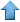Precision RecruitingData Mining Contest Math Jobs * Site Map
 [ Home ] [ Finance ] [ Web Audit ] [ Consulting ]

These are difficult mathematical questions. They are arising from real applications such as fraud detection, arbitrage and scoring systems. If you have interesting answers to any questions, feel free to email us your comments or solution. The best answers will be published here. Companies and Organizations interested in submitting problems should E-mail us.

### Approximate Solutions to Linear Regression Problems

Here we assume that we have a first order solution to a regression problem, in the form

Y = Σ wi Ri,

where Y is the response, wi are the regression coefficients, and Ri are the independent variables. The number of variables is very high, and the independent variables are highly correlated. We want to improve the model by considering a second order regression of the form

Y = Σ wi Ri + Σ wij cij mij Ri Rj ,

where

• cij = correlation between Ri and Rj
• wij = |wiwj|0.5 x sgn(wiwj)
• mij are arbitrary constants
In practice, some of the Ris are highly correlated and grouped into clusters. These clusters can be identified by using a clustering algorithm on the cijs. For example, one could think of a model with two clusters A and B such as

Y = Σ wi Ri + mA ΣA wij cij Ri Rj + mB ΣB wij cij Ri Rj

where

• ΣA (resp. ΣB) are taken over all i < j belonging to A (resp. B)
• mij = mA (constant) if i, j belong to cluster A
• mij = mB (constant) if i, j belong to cluster B
An interesting case occurs when the cluster structure is so strong that
• |cij| = 1 if i and j belong to the same cluster (either A or B)
• cij = 0 otherwise
This particular case results in

mA = 4 / [1 + (1+8kA)0.5]
mB = 4 / [1 + (1+8kB)0.5]

where kA= ΣA |cij| and kB= ΣB |cij|.

Question

If the cluster structure is moderately strong, with the correlations cij close to 1, -1 or 0, how accurate is the above formula involving kA and kB? Here we assume that the wis are known or approximated. Typically, wi is a constant or wi is a simple function of the correlation between Y and Ri.

Alternate Approach

Let us consider a simplified model involving one cluster, with mij = constant = m. For instance, the unique cluster could consist of all variables i, j with |cij| > 0.70. The model can be written as

Y = Σ wi Ri + m Σ wij cij Ri Rj.

We want to find m that provides the best improvement over the first order model, in terms of residual error. The first order model corresponds to m = 0. Let us introduce the following notations:

• W = Σ wij cij Ri Rj,
• V = W - u, where u = average(W) (Thus V is the centered W, with mean 0),
• S= Σ wi Ri. (average(S) = average(Y) by construction)
Without loss of generality, let us consider the slightly modified (centered) model

Y = S + m V.

Then

m = [ Transposed(V) x (Y-S) ] / [ Transposed(V) x V ],
where
• Y, S, and V are vectors with n rows,
• n is the number of observations.
Further Improvements

The alternate approach could be incorporated in an iterative algorithm, where at each step a new cluster is added. So at each step we would have the same computation for m, optimizing the residual error on

Y = S + m V.

However this time, S would contain all the clusters detected during the previous step, and V would contain the new cluster being added to the model.Data Mining • Machine Learning • Analytics • Quant • Statistics • Econometrics • Biostatistics • Web Analytics • Business Intelligence • Risk Management • Operations Research • AI • Predictive Modeling • Actuarial Sciences • Statistical Programming • Customer Insight • Data Modeling • Competitive Intelligence • Market Research • Information Retrieval • Computer Science • Retail Analytics • Healthcare Analytics • ROI Optimization • Design Of Experiments • Scoring Models • Six Sigma • SAS • Splus • SAP • ETL • SPSS • CRM • Cloud Computing • Electrical Engineering • Fraud Detection • Marketing Databases • Data Analysis • Decision Science • Text Mining